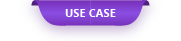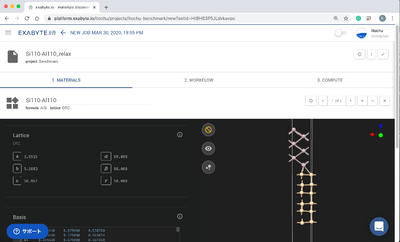In the field of semiconductors, research on metal / semiconductor interfaces is also important, so reports using first-principles calculations have been made.

# Calculation of Schottky barrier by silicon-aluminum interface model

In the field of semiconductors, research on metal / semiconductor interfaces is also important, so reports using first-principles calculations have been made. Therefore, here we will introduce how to create an interface model from two crystal structures and calculate the Schottky barrier. Here is a simple example made with the silicon and aluminum models. Since it is better to calculate by removing the periodic boundary of the Z axis, the ESM method is used.

#### 1. 1. Interface model creation

Create an interface model using Advance / NanoLabo based on the lattice constant and the structure-optimized crystal structure. NanoLabo makes it easy to create interface models because it does the proportions of each grid, matching calculations, and even simple interface optimization.

Export the structure created by NanoLabo to a CIF file and upload it to Exabyte for calculation.

#### 2. 2. Structural optimization

Structural optimization with Quantum ESPRESSO can be easily done by using the workflow already prepared.

#### 3. 3. Model creation for calculation by ESM method

Create the structure used in the ESM method based on the optimized structure. The structure used in ESM is not a normal structure, but Exabyte.io makes it easy to model an ESM.

#### 4. ESM method

By using ESM calculation, physical properties such as work functions and Schottky barriers are required with high accuracy, but input settings and visualization are required. Exabyte.io already has it, so you don't need to make complicated calculation settings.